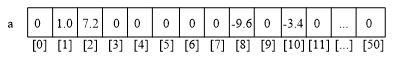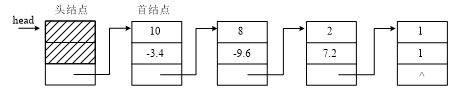数据结构实践——链表：多项式求和

【项目 - 多项式求和】
用单链表存储一元多项式，并实现两个多项式的加法。

1、存储多项式的数据结构
多项式的通式是pn(x)=anxn+an1xn1+...+a1x+a0$p_n(x)=a_nx^n+a_{n-1}x^{n-1}+...+a_1x+a_0$。n次多项式共有n+1项。直观地，可以定义一个数组来存储这n+1个系数。以多项式p(x)=3.4x109.6x8+7.2x2+x$p(x)=-3.4x^{10}-9.6x^8+7.2x^2+x$为例，存储这个多项式的数组如下图：可以看出，这种方案适合对某些多项式的处理。但是，在处理一些次数高但项数少的多项式时，存在浪费空间的现象，会有很多闲置的0。
可以使用如下定义的单链表结构存储多项式：链表中的每一个结点是多项式中的一项，结点的数据域包括指数和系数两部分，由指针域连接起多项式中的各项。
 typedef struct pnode //定义单链表结点类型，保存多项式中的一项，链表构成多项式 { double coef; //系数，浮点数 int exp; //指数，正整数* struct pnode *next; //指向下一项的指针 } PolyNode; 
用于表示多项式的链表将如下图所示，在建立多项式的链表时，已经令结点按指数由大到小的顺序排列。2、多项式加法在链表存储结构下的实现
链表存储结构下，多项式加法的实现 在如上定义的单链表存储结构基础上，讨论实现多项式加法的算法。
（1）若两个多项式中当前结点的指数值相同，则它们的系数相加，结果保存到p1结点，并将p2结点删除。如果相加后的系数不为0，p1指向第一个多项式的下一个结点，准备随后的工作，否则，不保存系数为0的项，将当前p1结点删除。
（2）当两个多项式中对应结点的指数值不相等时，若p1指向的结点的指数大，则p1简单地指向下一结点即可；而p2指向的结点大时，需要将p2结点插入到p1前，然后p2再重新指回到第二个多项式中的下一结点，继续进行处理。
（3）检测过程直到其中的任一个链表结束。若p1不为空，第一个多项式中的剩余项已经在链表中，不作处理，如果p2不为空，只需要将p2链接到相加后的第一个多项式末尾。
上面的讨论假设多项式链表中，已经按指数由大到小排序，在加法之前，采取多种手段保证这一前提成立。

［[参考解答]］

#include <stdio.h>
#include <malloc.h>
#define MAX 20          //多项式最多项数
typedef struct      //定义存放多项式的数组类型
{
double coef;        //系数
int exp;            //指数
} PolyArray;

typedef struct pnode    //定义单链表结点类型，保存多项式中的一项，链表构成多项式
{
double coef;        //系数
int exp;            //指数
struct pnode *next;
} PolyNode;

void DispPoly(PolyNode *L)  //输出多项式
{
bool first=true;        //first为true表示是第一项
PolyNode *p=L->next;
while (p!=NULL)
{
if (first)
first=false;
else if (p->coef>0)
printf("+");
if (p->exp==0)
printf("%g",p->coef);
else if (p->exp==1)
printf("%gx",p->coef);
else
printf("%gx^%d",p->coef,p->exp);
p=p->next;
}
printf("\n");
}

void DestroyList(PolyNode *&L)  //销毁单链表
{
PolyNode *p=L,*q=p->next;
while (q!=NULL)
{
free(p);
p=q;
q=p->next;
}
free(p);
}

void CreateListR(PolyNode *&L, PolyArray a[], int n) //尾插法建表
{
PolyNode *s,*r;
int i;
L=(PolyNode *)malloc(sizeof(PolyNode)); //创建头结点
L->next=NULL;
r=L;                        //r始终指向终端结点,开始时指向头结点
for (i=0; i<n; i++)
{
s=(PolyNode *)malloc(sizeof(PolyNode));//创建新结点
s->coef=a[i].coef;
s->exp=a[i].exp;
r->next=s;              //将*s插入*r之后
r=s;
}
r->next=NULL;               //终端结点next域置为NULL
}

{
if (p!=NULL)                //若原单链表中有一个或以上的数据结点
{
r=p->next;              //r保存*p结点后继结点的指针
p->next=NULL;           //构造只含一个数据结点的有序表
p=r;
while (p!=NULL)
{
r=p->next;          //r保存*p结点后继结点的指针
while (q->next!=NULL && q->next->exp>p->exp)
q=q->next;      //在有序表中找插入*p的前驱结点*q
p->next=q->next;    //将*p插入到*q之后
q->next=p;
p=r;
}
}
}

void Add(PolyNode *ha,PolyNode *hb,PolyNode *&hc)  //求两有序集合的并，完成加法
{
PolyNode *pa=ha->next,*pb=hb->next,*s,*tc;
double c;
hc=(PolyNode *)malloc(sizeof(PolyNode));        //创建头结点
tc=hc;
while (pa!=NULL && pb!=NULL)
{
if (pa->exp>pb->exp)
{
s=(PolyNode *)malloc(sizeof(PolyNode)); //复制结点
s->exp=pa->exp;
s->coef=pa->coef;
tc->next=s;
tc=s;
pa=pa->next;
}
else if (pa->exp<pb->exp)
{
s=(PolyNode *)malloc(sizeof(PolyNode)); //复制结点
s->exp=pb->exp;
s->coef=pb->coef;
tc->next=s;
tc=s;
pb=pb->next;
}
else                //pa->exp=pb->exp
{
c=pa->coef+pb->coef;
if (c!=0)       //系数之和不为0时创建新结点
{
s=(PolyNode *)malloc(sizeof(PolyNode)); //复制结点
s->exp=pa->exp;
s->coef=c;
tc->next=s;
tc=s;
}
pa=pa->next;
pb=pb->next;
}
}
if (pb!=NULL) pa=pb;    //复制余下的结点
while (pa!=NULL)
{
s=(PolyNode *)malloc(sizeof(PolyNode)); //复制结点
s->exp=pa->exp;
s->coef=pa->coef;
tc->next=s;
tc=s;
pa=pa->next;
}
tc->next=NULL;
}

int main()
{
PolyNode *ha,*hb,*hc;
PolyArray a[]= {{1.2,0},{2.5,1},{3.2,3},{-2.5,5}};
PolyArray b[]= {{-1.2,0},{2.5,1},{3.2,3},{2.5,5},{5.4,10}};
CreateListR(ha,a,4);
CreateListR(hb,b,5);
printf("原多项式A:   ");
DispPoly(ha);
printf("原多项式B:   ");
DispPoly(hb);
Sort(ha);
Sort(hb);
printf("有序多项式A: ");
DispPoly(ha);
printf("有序多项式B: ");
DispPoly(hb);
}